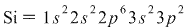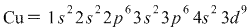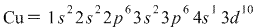# Give the ground-state electron configuration for silicon (Si)

Give the ground-state electron configuration for silicon (Si).
Give the actual ground-state electron configuration for copper (Cu).
Express the complete electron configuration using superscripts where appropriate. For example,the configuration for Li would be entered as 1s^22s^1.

To find the electron configuration for silicon, we first look atthe periodic table to see how many protons silicon has - 14protons. Since the problem asks for ground state, we know there areas many electrons as protons - thus, we want to express 14electrons.

Now we simply place the 14 electrons into their correct orbitals.Keeping in mind Aufbau’s principle (electrons go in lower-energyorbitals first), we start with 1s and move to 2s, 2p, 3s, andfinally 3p. Remember that s orbitals can hold 2 electrons while porbitals hold 6. Thus we fill in the orbitals in order until wereach 14:As you can see, the superscripts (denoting the number of electronsin each orbital) add up to 14.

Now let’s do copper. We see that copper has 29 protons, and it’sagain ground-state so we want 29 electrons. The orbitals after 3pare 4s and then 3d (remember that 3d comes before 4s!). Thus, onewould expect the ground state configuration of copper to bethis:However, there is a reason the problem asks for the ACTUALconfiguration of copper. This is because transition metals ingroups 6 and 11 (the columns containing Cr and Cu) prefer to have ahalf-filled or completely filled 3d orbital instead of a 3d orbitalwith 4 or 9 electrons. To accomplish this, one of the electronsfrom the 4s orbital actually moves up to 3d, leaving 4shalf-filled. This is just one of those exceptions you will have tomemorize.

So, the actual ground state configuration of copper is: